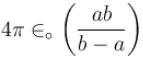Courses

# Capacitance MCQ Level - 1

## 10 Questions MCQ Test Basic Physics for IIT JAM | Capacitance MCQ Level - 1

Description
This mock test of Capacitance MCQ Level - 1 for Physics helps you for every Physics entrance exam. This contains 10 Multiple Choice Questions for Physics Capacitance MCQ Level - 1 (mcq) to study with solutions a complete question bank. The solved questions answers in this Capacitance MCQ Level - 1 quiz give you a good mix of easy questions and tough questions. Physics students definitely take this Capacitance MCQ Level - 1 exercise for a better result in the exam. You can find other Capacitance MCQ Level - 1 extra questions, long questions & short questions for Physics on EduRev as well by searching above.
QUESTION: 1

### The effective capacitance between A and B is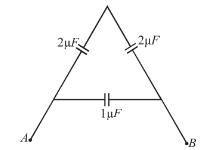Solution: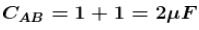QUESTION: 2

### Time constant of a C-R circuit is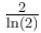second. Capacitor is discharged at time t = 0. The ratio of charge on the capacitor at time t = 2s and t = 6s is

Solution: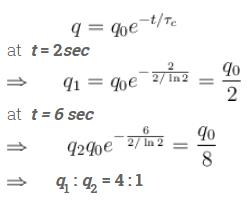The correct answer is: 4 : 1

QUESTION: 3

### Four plates are arranged as shown in the diagram. If area of each plate is A and the distance between two neighboring parallel plates is d, then the capacitance of this system will be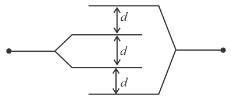Solution: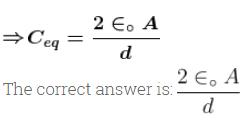QUESTION: 4

Force acting upon a charged particle kept between the plates of a charged condenser is F. If one of the plate of the condenser is removed, force acting on the same particle becomes

Solution: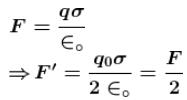QUESTION: 5

In the combination shown in the figure, the ideal voltmeter reading will be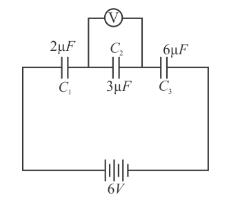Solution: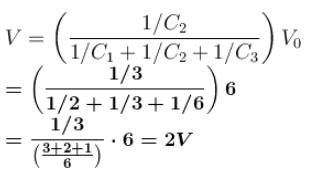QUESTION: 6

A parallel plate capacitor of plate area A and separation d is filled with two materials each of thickness d/2 and dielectric constants ∈1 and ∈2 respectively. The equivalent capacitance will be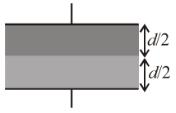Solution: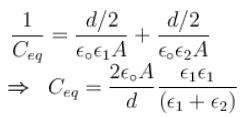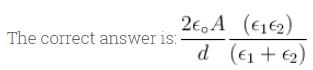QUESTION: 7

For the circuit shown in figure, the equivalent capacitance of the combination is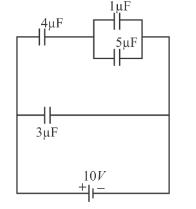Solution: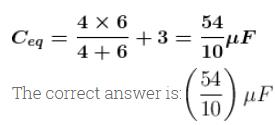QUESTION: 8

Between the plates of parallel plate condenser a plate of thickness t1 and dielectric constant k1 is placed in the rest of the space, there is another plate of thickness t2 and dielectric constant k2. The potential difference across the condenser will be

Solution: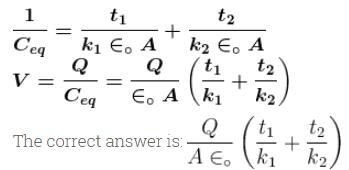QUESTION: 9

In the figure shown, the effective capacitance between the points P and Q, if each has capacitance C is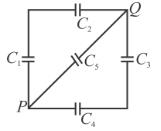Solution: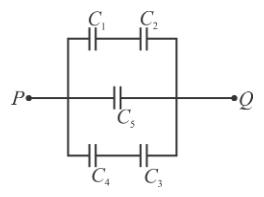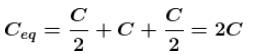QUESTION: 10

Two spherical conductor A and B of radii a and b (b > a) are placed concentrically in air. A is given a charge +Q while B is earthed. Then the equivalent capacitance of the system is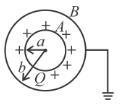Solution: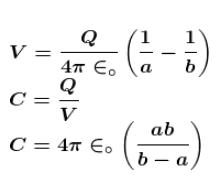The correct answer is: